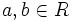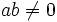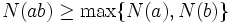# Multiplicatively monotone Euclidean norm

This article defines a property that can be evaluated for a Euclidean norm on a commutative unital ring

## Definition

A Euclidean norm is termed multiplicatively monotone if the norm of a nonzero product of two elements is at least equal to the norms of the elements. In symbols, if$N$ is a Euclidean norm on a commutative unital ring$R$, we say that$N$ is multiplicatively monotone if for any$a,b \in R$ such that$ab \ne 0$:$N(ab) \ge \max \{ N(a), N(b) \}$.